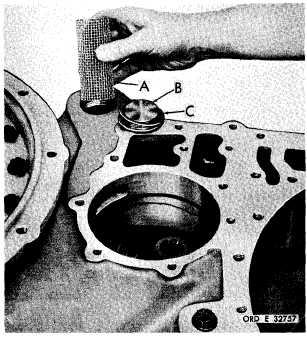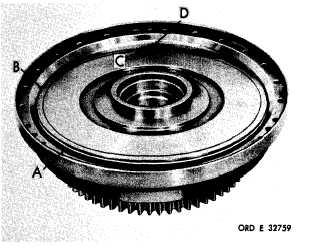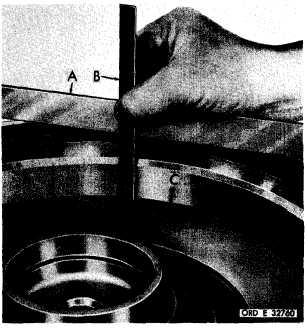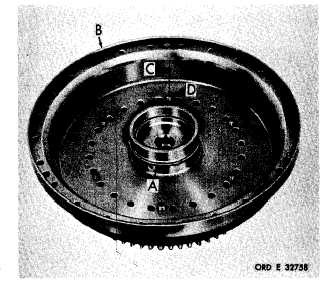Custom SearchC H A P    5,   SEC  X X X I I I A S S E M B LY PAR   228,    STEPS   1 - 14 Figure   338   (Step   11) Figure   340   (Step   13) Install   seal   and   expander   (A)   on   lockup   clutch piston   (B).   Place   an   index   mark   (C)   on   piston (B)   in   line   with   a   dowel   pinhole   on   the   opposite side.    Install    piston,    alining    index    marks    (C) and   (D). Install   scavenge   oil   pump   screen   (A)   and   plug (B)   with   gasket   (C). Figure   341   (Step   14) Figure   339   (Step   12) Install   hook-type   seal   ring   (A)   onto   hub   of   con- verter   pump   cover   (B).   Place   an   index   mark (C)   on   the   cover,   alined   with   dowel   pin   (D)   as s h o w n . Using   a   straightedge   (A)   and   scale   (B),   meas- ure   depth   of   lockup   clutch   piston   (C)   in   con- v e r t e r     c o v e r     ( D ) .     M e a s u r e m e n t     s h o u l d     r e ad a p p r o x i m a t e l y    2 - 1 / 1 6    i n c h e s    w h e n    p i s t o n    is p r o p e r l y    i n s t a l l e d. 1 8 8